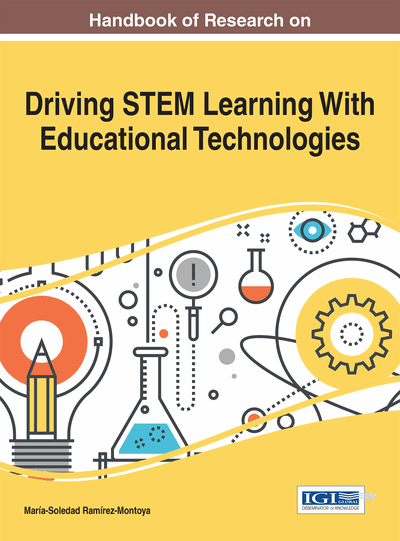# Didactic Sequences Teaching Mathematics for Engineers With Focus on Differential Equations

Luis Ramón Siero González (Instituto Politécnico Nacional, Mexico & Universidad Autónoma de Baja California, Mexico) and Avenilde Romo Vázquez (Instituto Politécnico Nacional, Mexico)
Copyright: © 2017 |Pages: 23
DOI: 10.4018/978-1-5225-2026-9.ch007
OnDemand PDF Download:
Available
\$37.50
No Current Special Offers

## Abstract

This investigation takes place in the Research Center for Applied Science and Advance Technology of the National Polytechnic Institute (CICATA-IPN) and in the School of Science of Engineering and Technology of the Autonomous University of Baja California (ECITEC-UABC), having the objective of designing a mathematical modeling activity for the training of future engineers in the subjects of differential equations, and circuits. Considering the Anthropological Theory of Didactics (ATD) implementing a methodology within the mentioned theory that allows the analysis of mathematical models in use, as basis for designing didactical activities, in order to create a link between these two subjects, showing the future engineers that the mathematics can be used to solve problems in an extra-mathematical context.
Chapter Preview
Top

## Introduction

This research was conducted at the Center for Research in Applied Science and High Technology at the National Polytechnic Institute (CICATA for their acronym in Spanish) of Mexico and the School of Science of Engineering and Technology (ECITEC for their acronym in Spanish) of the Autonomous University of Baja California. The objective of this work is to design modeling activities for a mathematical training of engineers. The design is based on mathematical modeling analysis on specialty training courses, focusing on circuits. This collaborative work involves electronic engineer and mathematicians that teach in the carriers of Aerospace engineering and mechatronics engineering, having an opportunity to analyze a real context of modeling; namely RC circuit within a pacemaker. This project was proposed on a differential equation course, because the students wanted to know where they could use the mathematics they were learning. This work considers elements of the anthropological theory of didactics proposing a methodology associated to this theory that allows the analysis of mathematical models in use based on the design of didactic activities.

The mathematical training of future engineers has been studied in different research in mathematics education (Bissell & Dillon 2000; Bissell 2002; Camarena, 1999; Kent & Noss 2000 & 2001; Macias 2012; Martínez 2014; Romo-Vázquez 2009; Soto 2013). These studies have shown that the teaching of mathematics in engineering education caters to particular needs. Pollak (1988) shows that these basic and advanced mathematical needs can be addressed from mathematical modeling, considering it has a basis for a new educational paradigm. In fact, mathematical modeling has been addressed in a variety of research in the discipline. The ICMI 14 study published in 2007 (Artaud, 2007) shows different theoretical perspectives that have been produced to study. In the introduction of this investigation a mathematical model is defined as follows:

A mathematical model consists of the extra- mathematical domain, D, of interest, some mathematical domain M, and a mapping from the extra-mathematical to the mathematical domain.

Meanwhile modeling is defined as:

Objects, relations, phenomena, assumptions, questions, etc. in D are identified and selected as relevant for the purpose and situation and are then mapped ~ translated - into objects, relations, phenomena, assumptions, questions, etc. pertaining to M. Within M, mathematical deliberations, manipulations and inferences are made, the outcomes of which are then translated back to D and interpreted as conclusions concerning that domain. (Niss, Blum & Galbrahit, 2007, p.4)

It could be said that this idea of modeling, conceived as a cycle that can be repeated several times, with some variations in different research. Bisell (2002) presents two modeling cycles that he calls rigid and flexible. However he points out that they are based on a platonic conception of modeling, assuming that every phenomenon can be modeled. He points out that the engineers do not build models whose solutions are well known and have been used on other occasions; they adapt and refine them. This leads to the question, how do engineers model in their specialty training and practice? Which are the mathematical models they use? Are these models being taught in their mathematical training?

## Key Terms in this Chapter

Type Task: An activity that is related with certain problems, and they are cataloged as mathematical and non-mathematical.

Didactical Activity: An activity that helps a student understand and comprehend a theme of a subject.

Technique: The manner in which someone can solve a Type task activity.

Technology: The component that justifies a technique.

Mathematical Modeling: A process that gives a result to a representation of a physical phenomenon using mathematics.

Praxeology: A structure that helps break down any human activity, and has four components: Type task, Techniques, Technologies and Theory.

Theory: The component that justifies and gives meaning to the technologies.

Didactical Sequence: An ensemble of didactical activities that help a student to comprehend a theme of a subject.

## Complete Chapter List

Search this Book:
Reset